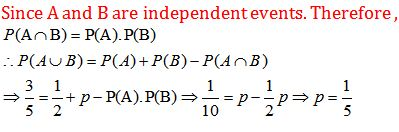# Topic: Probability (Test 1)

Topic: Probability
Q.1
The conditional probability of an event E, given the occurrence of the event F is given by
A. P(E|F)=P(EF)P(F),P(F)<0
B. P(E|F)=P(EF)P(F),P(F)0
C. P(  |   F)   =P(EF)P(F), P(F)0
D. P(E|F)=P(EF)P(E),P(F)0
Answer : Option C
Explaination / Solution:

The conditional probability of an event E, given the occurrence of the event F is given by :

Workspace
Report
Q.2
If P (A) = 0.8, P (B) = 0.5 and P(B|A) = 0.4, find P(A|B)
A. 0.68
B. 0.64
C. 0.66
D. 0.62
Answer : Option B
Explaination / Solution:

We have ,
P (A) = 0.8, P (B) = 0.5 and P(B|A) = 0.4Workspace
Report
Q.3
A dice is tossed once and even number has come up. The chance that it is either 2 or 4 is
A. 2/ 6
B. None of these
C. 4/ 9
D. 2/ 3
Answer : Option D
Explaination / Solution:

Total number of ways of getting even number is 3

Out of these 3 even number we have to get either 2 or 4 which can be done in 2 ways.

So required probability is 2/3

Workspace
Report
Q.4
The letters of the word ‘ ORIENTAL ‘ are arranged in all possible ways . The chance that the consonants and vowels occur alternately is
A. 2/ 35
B. None of these
C. 1/35
D. 1/70
Answer : Option C
Explaination / Solution:
No Explaination.

Workspace
Report
Q.5
Let A and B be two independent events. The probability that both A and B occur is  and the probability that neither A nor B occurs is . The respective probabilities of A and B are
A. 12and16
B. 1/3 and 1/4 or 1/4 and 1/3
C. none of thse
D. 16and12
Answer : Option B
Explaination / Solution:
No Explaination.

Workspace
Report
Q.6
One ticket is selected at random from 100 tickets numbered 00, 01, 02…, 99. Suppose S and T are the sum and product of the digits of the number on the ticket, then the probability of getting S = 7 and T = 0 is
A. 150
B. 219
C. 19100
D. 14
Answer : Option A
Explaination / Solution:
No Explaination.

Workspace
Report
Q.7
A cubical dice has 3 on three faces , 2 on two faces and 1 on the 6  face .It is tossed twice . The chance that both the tosses show an even number is
A. none of these.
B. 1/ 36
C. 1/4
D. 1/9
Answer : Option D
Explaination / Solution:
No Explaination.

Workspace
Report
Q.8

Given that the events A and B are such that P(A) =, P (A ∪ B) = and P(B) = p. Find p if they independent.

A. 12
B. 14
C. 13
D. 15
Answer : Option D
Explaination / Solution:Workspace
Report
Q.9
In answering a question on a multiple choice test, a student either knows the answer or guesses. Let  be the probability that he knows the answer and  be the probability that he guesses. Assuming that a student who guesses at the answer will be correct with probability  . What is the probability that the student knows the answer given that he answered it correctly?
A. 1113
B. 1213
C. 713
D. 913
Answer : Option B
Explaination / Solution:

Let are events that the student knows the answer and the student guesses respectively.Workspace
Report
Q.10
A coin is tossed three times, if E : head on third toss , F : heads on first two tosses. Find P(E|F)
A. 1/5
B. 1/2
C. 1/3
D. 2/3
Answer : Option B
Explaination / Solution:Workspace
Report